Smallest number multiplied to get perfect square

Chapter 6 Class 8 Squares and Square Roots
Concept wise

Let’s do this by examples

### Finds the smallest number multiplied by 32 to get a perfect square.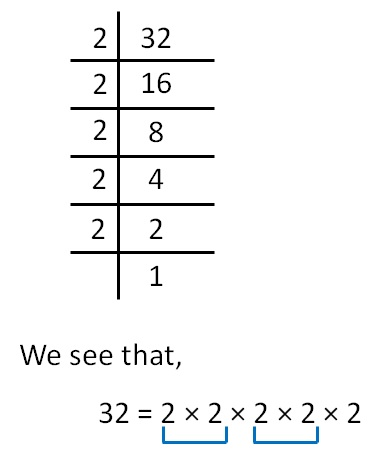Since 2 does not occur in pair,

we multiply by 2 to make it a pair

So, our number becomes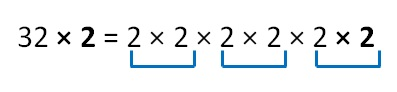Now, it becomes a perfect square.

So, we multiply 32 by 2 to make it a perfect square

### Finds the smallest number multiplied by 90 to get a perfect square.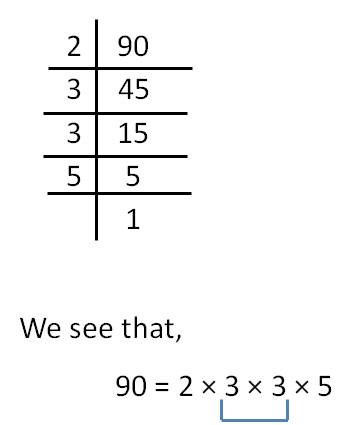Here,

2 & 5 do not occur in pairs

So, we multiply by 2 and 5 to make pairs

So, our number becomes

90 × 2 × 5 = 2 × 3 × 3 × 5 × 2 × 5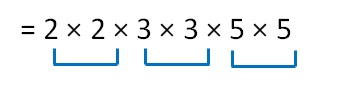Now, it becomes a perfect square.

So, we multiply 90 by 2 × 5

i.e. 10  to make it a perfect square

### Finds the smallest number multiplied by 120 to get a perfect square.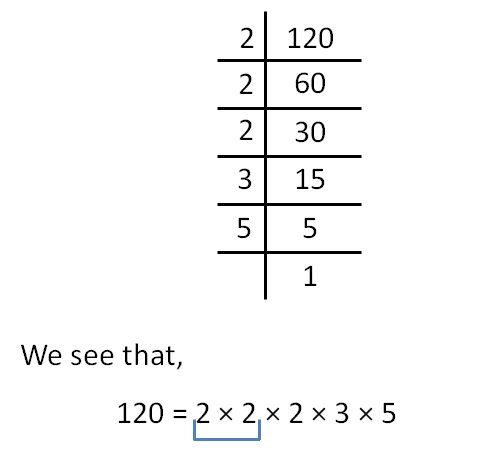Here,

2, 3 & 5 do not occur in pairs

So, we multiply by 2, 3 and 5 to make pairs

So, our number becomes

120 × 2 × 3 × 5 = 2 × 2 × 2 × 3 × 5 × 2 × 3 × 5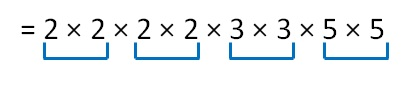Now, it becomes a perfect square.

So, we multiply 120 by 2 × 3 × 5

i.e. 30  to make it a perfect square

Get live Maths 1-on-1 Classs - Class 6 to 12